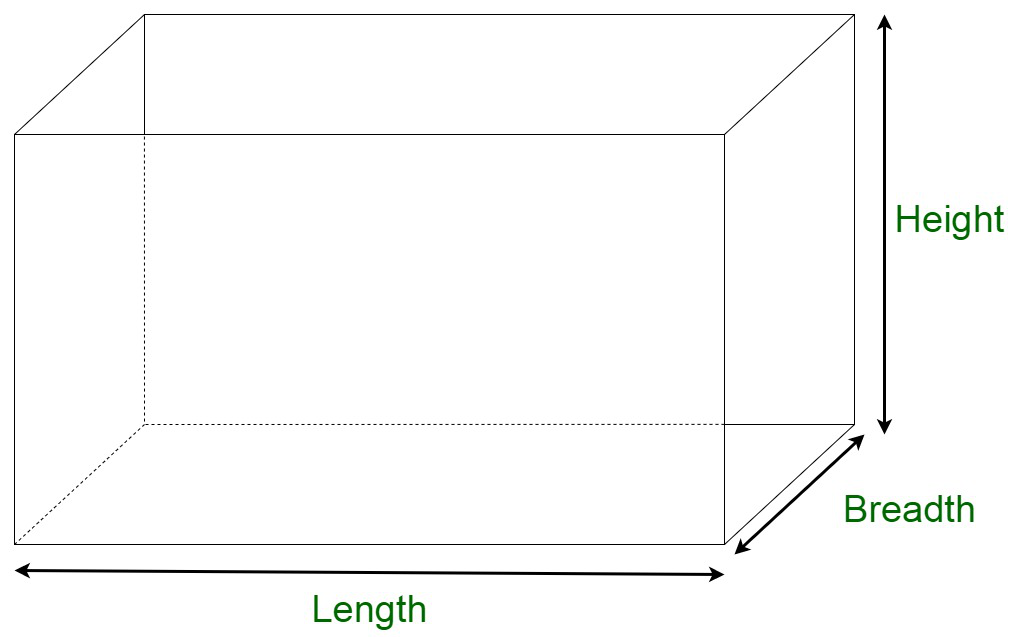How to find the volume of a cuboid?

• Last Updated : 21 Nov, 2021

Cuboid is a three-dimensional shape that is characterized by three dimensions, that is, length, width, and height. The cuboid shape has six sides called faces. Each of the faces of a cuboid is a rectangle, and all of a cuboid’s vertices are contained at right angles.Properties of a Cuboid

A cuboid is characterized by the following properties:

• A cuboid has 6 faces, 8 vertices, and 12 edges.
• All the angles are right angles.
• All the faces are rectangular in shape.
• Each face contains two diagonals.
• The opposite edges of a cuboid are parallel to each other.
• The dimensions of a cuboid are length, width, and height.

Volume of Cuboid

The volume of the cuboid can be considered as the measure of the space occupied within a cuboid. A cuboid is identified by the following three dimensions, that is, length, breadth, and height. The unit of volume of cuboid is given as the (unit)3.

Volume of Cuboid Formula

Let us assume the area of a rectangular sheet of paper to be ‘A’, and the height up to which they are stacked be ‘h’. In addition to this, let us assume the volume of the cuboid be ‘V’. Then, the volume of the cuboid is obtained by the multiplication of the base area and height.

The volume of cuboid = Base area × Height

The base area for cuboid = l × b

Hence, the volume of a cuboid, V = l × b × h = lbh

Steps to find the volume of the cuboid:

Step 1: All the dimensions of the cuboid are converted to a similar set to achieve consistency.

Step 2: The product of the length, breadth, and height of the cuboid is obtained.

Step 3: The unit is appended to the product.

Sample Questions

Question 1. Find the volume of cuboid with length = 50 cm, breadth = 30 cm, height = 15 cm?

Solution:

Here we have to find the volume of the cuboid with the given dimensions

Given:

Length (l) = 50 cm

Height (h) = 15 cm

As we know that,

Volume of cuboid = Length × Breadth × Height

Volume of cuboid = l × b × h

Volume of cuboid = 50 × 30 × 15

Volume of cuboid = 22500 cm3

Therefore,

Volume of the cuboid with length = 50 cm, breadth = 30 cm, height = 15 cm is 22500 cm3.

Question 2. Calculate the volume of the cuboidal warehouse with the following dimensions;

Length = 30 m

Height = 10 m

Solution:

Here we have to find the volume of the cuboidal warehouse with the given dimensions

Given:

Length (l) = 30 m

Height (h) = 10 m

As we know that,

Volume of cuboid = Length × Breadth × Height

Volume of cuboidal warehouse = l × b × h

Volume of cuboidal warehouse = 30 × 15 × 10

Volume of cuboid = 4500 m3

Therefore,

Volume of the cuboidal warehouse with length = 30 m, breadth = 15 m, height = 10 m is 4500 m3.

Question 3. Find the length of the cuboid volume is 750 cm3 and breadth is 10 cm, height is 5 cm?

Solution:

Here we have to find the length (l) of the cuboid

Given:

Volume of cuboid = 750 cm3

Height (h)  = 5 cm

As we know that

Volume of cuboid = Length × Breadth × Height

Volume of cuboid = l × b × h

⇒ 750 = l × 10 × 5

⇒ 750 = l × 50

⇒ l =⇒ l = 15 cm

Therefore,

Length of cuboid is 15 cm.

Question 4. Find the volume of cuboid when the length of the cuboid is four times the height and breadth is twice the height. The height of the cuboid is 10 m?

Solution:

Here we have to find the volume of the cuboid

Given:

Height (h) = 10 m

Length (l) = 4 × h = 4 × 10 = 40 m

Breadth (b) = 2 × h = 2 × 10 = 20 m

Now,

As we know that,

Volume of cuboid = Length × Breadth × Height

Volume of cuboid = l × b × h

Volume of cuboid = 40 × 20 × 10

Volume of cuboid = 8000 m3

Therefore,

Volume of cuboid is 8000 m3.

Question 5. Calculate the amount of air can be filled in a cuboidal box with length = 100 cm, breadth = 50 cm, height = 25 cm?

Solution:

Here we have to find the amount of air that can be filled in the cuboidal box.

Air that can be filled in the cuboidal box = Volume of the cuboidal box

Given:

Length (l) = 100 cm

Height (h) = 25 cm

As we know that,

Volume of cuboid = Length × Breadth × Height

Volume of cuboidal box = l × b × h

Volume of cuboidal box = 100 × 50 × 25

Volume of cuboidal box = 125000 cm3

Therefore,

The amount of air that can be filled in a cuboidal box is 125000 cm3.

My Personal Notes arrow_drop_up